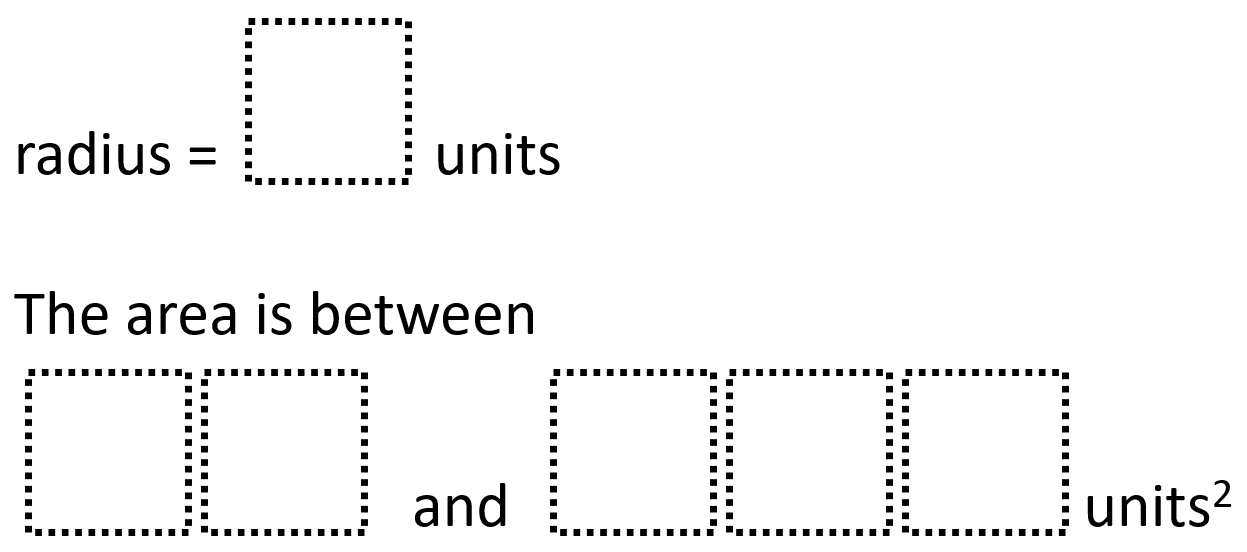# Circle Radius and Area 1

Directions: Using the digits 0 to 9 at most one time each, fill in the boxes to create two possible circles. You may reuse all the digits for each statement.### Hint

How many digits of precision does pi require for this problem?

There are many possible answers including:
radius = 5 units and the area is between 21 and 678 square units
radius = 9 units and the area is between 87 and 260 square units

Source: Robert Kaplinsky

## Two Step Inequality with Fractional Coeffcient

Directions: Using the digits 1 to 7 at most one time each, place a digit …

### One comment

1.Jennifer Howland

Could you provide further explanation on how to solve this problem? Thank you!